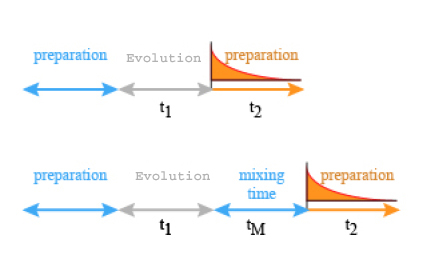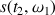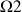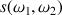# 2D NMR Basics

$$\newcommand{\vecs}{\overset { \rightharpoonup} {\mathbf{#1}} }$$ $$\newcommand{\vecd}{\overset{-\!-\!\rightharpoonup}{\vphantom{a}\smash {#1}}}$$$$\newcommand{\id}{\mathrm{id}}$$ $$\newcommand{\Span}{\mathrm{span}}$$ $$\newcommand{\kernel}{\mathrm{null}\,}$$ $$\newcommand{\range}{\mathrm{range}\,}$$ $$\newcommand{\RealPart}{\mathrm{Re}}$$ $$\newcommand{\ImaginaryPart}{\mathrm{Im}}$$ $$\newcommand{\Argument}{\mathrm{Arg}}$$ $$\newcommand{\norm}{\| #1 \|}$$ $$\newcommand{\inner}{\langle #1, #2 \rangle}$$ $$\newcommand{\Span}{\mathrm{span}}$$ $$\newcommand{\id}{\mathrm{id}}$$ $$\newcommand{\Span}{\mathrm{span}}$$ $$\newcommand{\kernel}{\mathrm{null}\,}$$ $$\newcommand{\range}{\mathrm{range}\,}$$ $$\newcommand{\RealPart}{\mathrm{Re}}$$ $$\newcommand{\ImaginaryPart}{\mathrm{Im}}$$ $$\newcommand{\Argument}{\mathrm{Arg}}$$ $$\newcommand{\norm}{\| #1 \|}$$ $$\newcommand{\inner}{\langle #1, #2 \rangle}$$ $$\newcommand{\Span}{\mathrm{span}}$$$$\newcommand{\AA}{\unicode[.8,0]{x212B}}$$

## Durations

The 2D NMR experiment belongs as well to the Fourier transform spectroscopy than to the impulsion one and relies on a sequence of three time intervals: preparation, evolution and detection (3). In some experiment another time interval is added before the detection: the mixing time ( Figure $$\PageIndex{1}$$).Figure $$\PageIndex{1}$$: Scheme for time pulse in a 2 D NMR experiment (3)

### The preparation time

Upon the preparation time the spin system under study is firstly prepared, for example it is submitted either to a decoupling experiment or just to a transverse magnetization by the means of a 90° impulsion. It allows the excited nuclei to get back their equilibrium state between two successively executed pulse sequence (5)

### The evolution time t1

During the evolution time $$t_1$$, the spin system is evolving under the effect of different factors, each coherence evolves at its own characteristic frequency as a function of the chemical shift and of the scalar coupling of the corresponding nucleus.

### The Mixing time

It is made of a pulse sequence which achieves coherence transfers in such a way that different frequencies can be correlated.

### The detection time

The acquisition of the modulated signal takes place during the detection period. The sequence we just described does not constitute by itself a 2D NMR experiment.

## JEENER

The idea of Jeener consists in the stepwise increasing of the evolution time tl. This will allow to get an NMR signal under the aspect of a sampling of free precession signals of the s(t2) type. These FID will differ from each other only by the tl period duration written under a matricial form s(tl ,t2) (5). The tl delay is the time between the first and the second pulse ( Figure $$\PageIndex{2}$$).Figure $$\PageIndex{2}$$: Sampling of signals of free precession of the type s(t2)

The first Fourier transform as a function of tl gives us an interferogram of the form( Figure $$\PageIndex{3}$$).Figure $$\PageIndex{3}$$: Interferogram of the form $$\left(t_{2}, \omega_{1}\right)$$

A second Fourier transform, versus the second variable t2, gives an NMR spectrum with two frequencies dimensions F1 and F2 (Figure $$\PageIndex{3}$$). The result of this two fold Fourier transform does not get two spectraandbut only one spectrum as a function of two independent frequencies, exhibiting a peak with the coordinates. Thus, an alimentation evolving with the frequencyin the time course t1 has been converted in another coherence evolving with the frequencyduring the period t2.Figure $$\PageIndex{4}$$: NMR spectrum in two dimensions following the second Fourier transform(4).

This double Fourier transform in both dimension yields thus a matrix. ( Figure $$\PageIndex{3}$$ ).

2D NMR Basics is shared under a CC BY 4.0 license and was authored, remixed, and/or curated by Marc Bria.